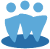## Relative Value (RV) and Projected Relative Value on SSG do not match RV and Projected RV on other reports.

The Relative Value (RV) and Projected Relative Value figures on the back of the SSG in Toolkit 6 may not match the RV and Projected RV figures on other reports, because the SSG uses a different formula than the other reports in Toolkit 6.

The Relative Value (RV) figure in section 3 of the SSG is based on the Average High and low P/E figures that appear on line 6 of section 3. The RV figure on other reports use variations of the standard formula.

Here is the basic formula for calculating Relative Value (RV):
RV = (Current PE / Average 5 Year PE) * 100
On the back side of the SSG, the Average 5 Year PE formula is:
(Avg5YrHighPE + Avg5yrLowPE) / 2
With that in mind, the formula written out in long-form looks like this:
RV = (Current PE / ((Avg5YrHighPE + Avg5yrLowPE) / 2)) * 100

Projected Relative Value on the back of the SSG changes the formula slightly:
Projected RV = (Current Price / (PE Ratio * (1 + Projected EPSGrowth/100))) / Average 5 Year PE * 100
PE Ratio is calculated as (Current Price/Current P/E)
Average 5 Year PE in this formula is the same as in the regular RV formula.

The terms Relative Value and Projected Relative Value also show up other reports, such as the Quarterly Trend Report (PERT), and on portfolio reports, such as the Summary, Offense, Overview, and Offense Alert. These reports use two slight variations on the Relative Value and Projected Relative Value formulas from the SSG.

The following formula is used for Projected RV on the PERT and Portfolio Offense report, as well as for standard RV on the Portfolio Summary, and Portfolio Overview:
Projected PE/Average Selected 5 Year P/E * 100.
Here, Projected PE = (Current Price / (PERatio * (1 + Projected EPSGrowth/100)))
Also, In this formula, Average Selected 5 Year P/E is (Selected High P/E from section 4 + Selected Low P/E from section 4) / 2
This is different from the Average 5 Year P/E used on the back of the SSG, which only uses the Average P/E from section 3.

The final variation on the formula is used as Relative Value on the Portfolio Offense report, and on the Portfolio Alerts window:
CurrentPE / Average Selected 5 Year P/E * 100
Average Selected 5 Year P/E here is the same as in the previous formula, where it uses selected High and Low P/E figures from section 4.